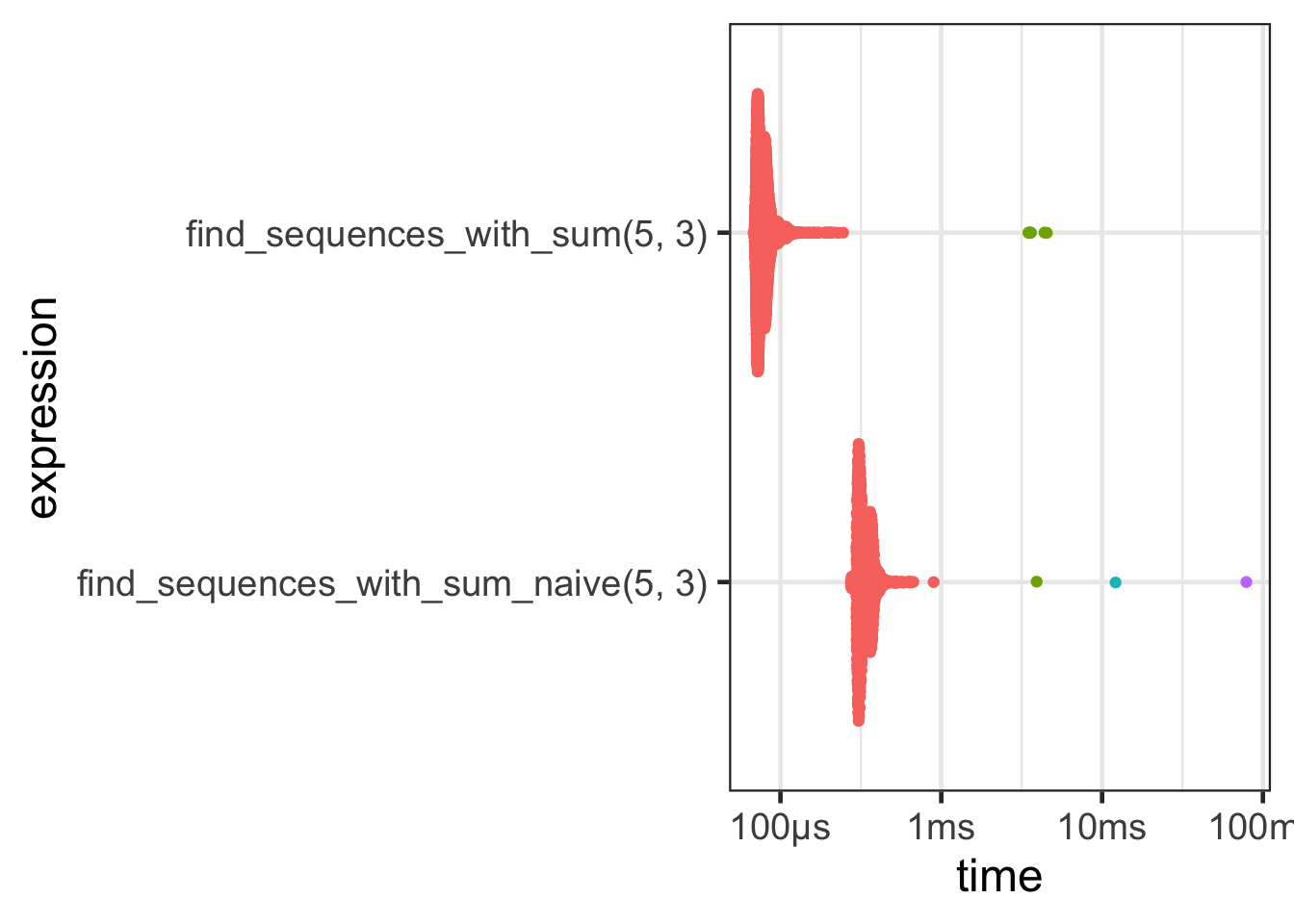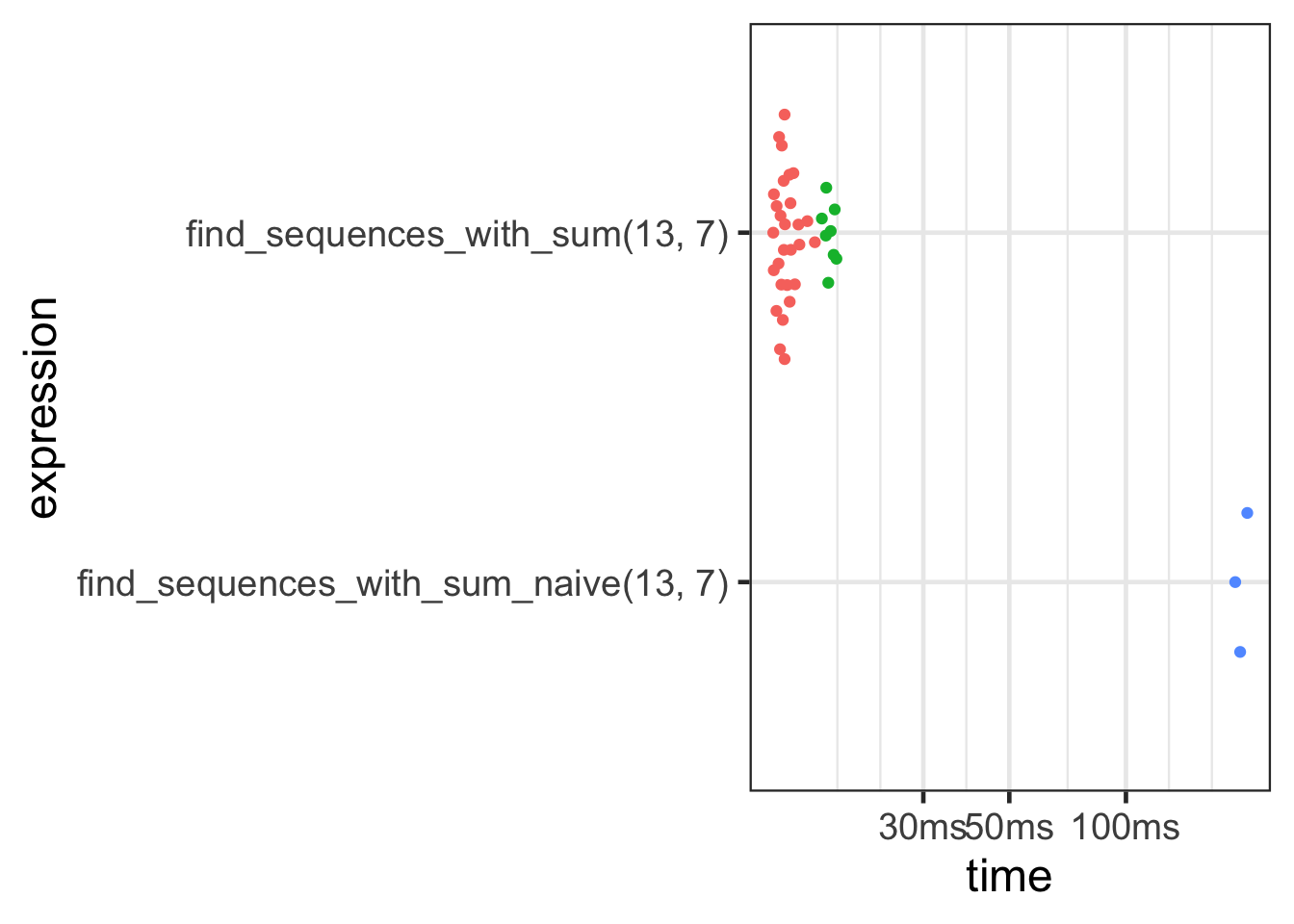# Find all sequences of a given length with the given sum

## Problem

This problem popped up as a necessary step to solving a type of puzzle (which I’ll post about soon).

• From the set of all positive (non-zero) integers, find all the sequences of the specified length which have the given sum.
• Need all orderings
• sums usually range from 10 to 30
• lengths usually range from 2 to 10

## Example: All the length=3 vectors which sum to 5 (one sequence per row)

``````     [,1] [,2] [,3]
[1,]    1    1    3
[2,]    1    2    2
[3,]    1    3    1
[4,]    2    1    2
[5,]    2    2    1
[6,]    3    1    1``````

## Naive solution - generate all sequences, then filter

• Use expand grid to expand all possible sequences.
• Then filter where `rowSums()` is equal to the required `target_sum`
• Scales really badly as the sum and length targets get bigger
``````find_sequences_with_sum_naive <- function(target_sum, target_length) {
res <- do.call(expand.grid, replicate(n=target_length, seq(target_sum - target_length + 1L), simplify = FALSE))
res[rowSums(res) == target_sum,]
}

find_sequences_with_sum_naive(5, 3)``````
``````   Var1 Var2 Var3
3     3    1    1
5     2    2    1
7     1    3    1
11    2    1    2
13    1    2    2
19    1    1    3``````

## Iterative solution with back-tracking

• build up vectors one digit at a time
• stop if the required length or sum is exceeded and try a different sequence
``````find_sequences_with_sum <- function(target_sum, target_length, current_vec=c(), N=0L, S=0L) {

stopifnot(target_sum >= target_length)

#~~~~~~~~~~~~~~~~~~~~~~~~~~~~~~~~~~~~~~~~~~~~~~~~~~~~~~~~~~~~~~~~~~~~~~~~~~~
# If we're at the right length, have we found a solution?
#~~~~~~~~~~~~~~~~~~~~~~~~~~~~~~~~~~~~~~~~~~~~~~~~~~~~~~~~~~~~~~~~~~~~~~~~~~~
if (N == target_length) {
if (S == target_sum) {
return(list(current_vec))
} else {
return()
}
}

#~~~~~~~~~~~~~~~~~~~~~~~~~~~~~~~~~~~~~~~~~~~~~~~~~~~~~~~~~~~~~~~~~~~~~~~~~~~
# Limit the choices in the next iteration to just ones that are possible
#~~~~~~~~~~~~~~~~~~~~~~~~~~~~~~~~~~~~~~~~~~~~~~~~~~~~~~~~~~~~~~~~~~~~~~~~~~~
max_choice <- (target_sum - S) - (target_length - N) + 1L
choices <- 1:max_choice

#~~~~~~~~~~~~~~~~~~~~~~~~~~~~~~~~~~~~~~~~~~~~~~~~~~~~~~~~~~~~~~~~~~~~~~~~~~~
# For each choice, add it to the current_vec and see if that is a pathway
# to a solution
#~~~~~~~~~~~~~~~~~~~~~~~~~~~~~~~~~~~~~~~~~~~~~~~~~~~~~~~~~~~~~~~~~~~~~~~~~~~
solutions <- list()
for (new_val in choices) {
this_solution <- find_sequences_with_sum(target_sum, target_length, c(current_vec, new_val), N=N+1L, S=S+new_val)
solutions <- c(solutions, this_solution)
}

solutions
}

do.call(rbind, find_sequences_with_sum(5, 3)) ``````
``````     [,1] [,2] [,3]
[1,]    1    1    3
[2,]    1    2    2
[3,]    1    3    1
[4,]    2    1    2
[5,]    2    2    1
[6,]    3    1    1``````

## Quick benchmark

• For small problems, results are roughly similar.

### Small exampleexpression median itr/sec mem_alloc
find_sequences_with_sum_naive(5, 3) 321.3µs 2956.288 64.9KB
find_sequences_with_sum(5, 3) 77.4µs 12581.641 0B

### Medium-sized example

• Once the problem becomes moderately large, the back-tracking solution (to no-one’s surprise) is faster and uses less memory.
• in this case, ~3x as fast and ~1000x less memoryexpression median itr/sec mem_alloc
find_sequences_with_sum_naive(13, 7) 197.1ms 5.048857 104MB
find_sequences_with_sum(13, 7) 13.4ms 70.538884 142KB

## Summary

• Use the back-tracking solution